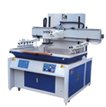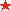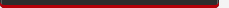<% set rs=conn.execute("select content as content from sy_basic_info where id=5") if not rs.eof then response.write(rs("content")) else response.write("constructing now.....") end if rs.close set rs=nothing %><% set rs=conn.execute("select content as content from sy_basic_info where id=7") if not rs.eof then response.write(rs("content")) else response.write("constructing now.....") end if rs.close set rs=nothing %>
<% set rs=conn.execute("select * from sy_products order by ptjnum,plist desc") z=0 do while not rs.eof and z < 4 z=z+1 %>
<%=rs("pname")%>

<%=cutstr(nohtml(rs("pcontent")),60)%>" style="color:#000;">read more
<% rs.movenext loop set rs=nothing %>
more+联系方式<% set rs=conn.execute("select content as content from sy_basic_info where id=6") if not rs.eof then response.write(rs("content")) else response.write("constructing now.....") end if rs.close set rs=nothing %>